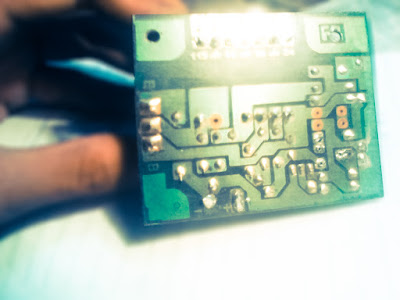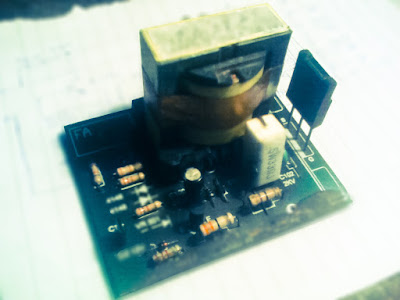# Universal switching power supply for TV

The schematic above can be used for supply on color TV for all type TV circuit. But we need to know how much voltage needed by the circuit of color Television. To set the output voltage, the setting on VR1 1K ohm. Required input voltage 300V DC which is already on TV circuit, so we just take a 300V dc voltage existing on the TV circuit, and for a circuit supply voltage on our TV cut the line PCB and connected with this  universal switching power supply.For the transformer we can use the TV regulator transformer.
Part List :
• R1 = 33R 5W
• R2 = 330R 1W
• R3 = 2K2
• R4 = 47K
• R5 = 2K7
• R6 = 22K
• R7 = 390K
• R8 = 47R 1W
• C1 = 100uF 25V
• C2 = 1n2 2KV
• C3 = 18n
• C4 = 18n
• D1 = 1N4148
• D2 = 1N4148
• D3 = ZD 12V
• D4 = 1N4007
• D5 = 1N4007
• VR1 = 1K
• ZD1 = Zener 6V
• T1    = TV regulator transformer
• Q1   = K2847
• Q2   = C2328APCB lineFinishes circuit

#### 1 comment

1.Whats uρ this іs kindа of off
toрic but I ωаs wonderіng if blogs use WΥSӏWΥG
eԁіtorѕ oг if you havе to manually сode with HTML.
I'm starting a blog soon but have no coding knowledge so I wanted to get guidance from someone with experience. Any help would be enormously appreciated!
Also see my web site: Reprise Automobile